# Fractals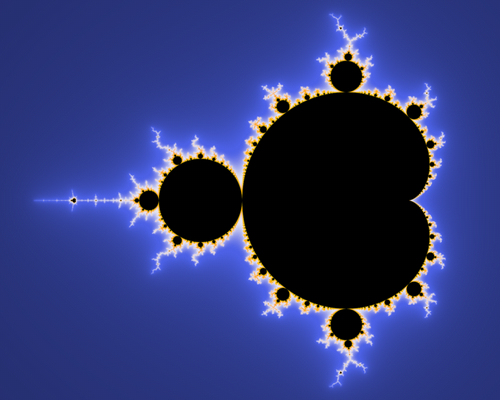# Fractals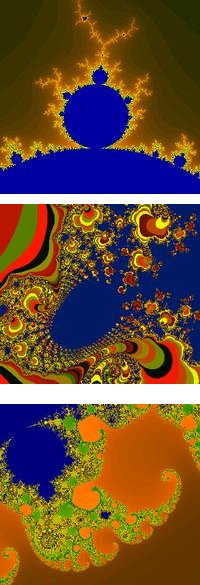Fractals feature fine detail at arbitrary scales.

They are usually highly irregular.

They exhibit some degree of self-similarity.

They can be mathematically defined.

# Mandelbrot set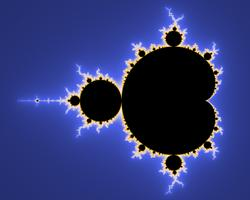Classic fractal image, described by Benoit Mandelbrot.

The set is those points (c) in the complex plane for which the formula:

```    z = z2 + c
```
remains finite when applied iteratively.

This can be estimated by

```    z = c
for i in range(0, maxIterations):
z = z*z + c
if abs(z) > 2: return False
return True
```

Sample code: glmandelbrot.py

# Other Fractals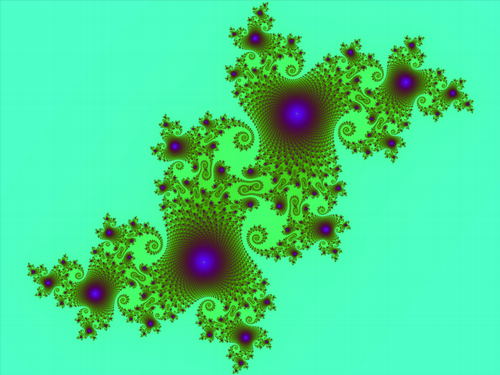Julia Set

# Other Fractals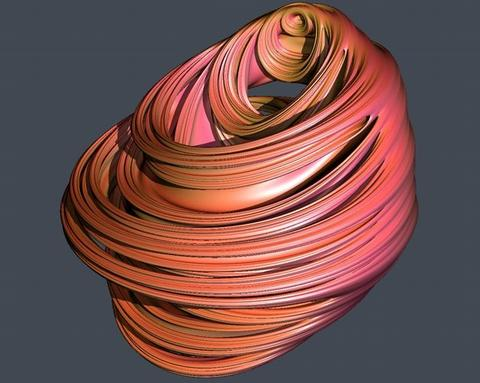Quaternion (4D) Julia Set

# Other FractalsKoch Curve

# Fractal terrain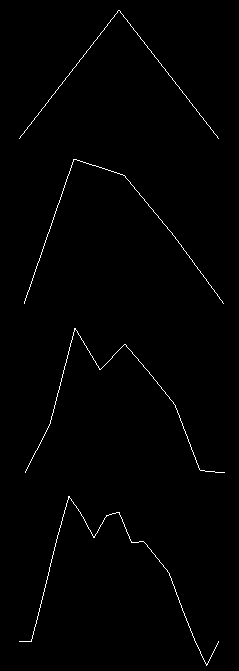Fractal terrain can be generated in different ways, some using statistical filtering of noise.

Another, simple method is "midpoint subdivision".

Start with a basic terrain model, and add detail - split each edge at its midpoint, and move the point up or down randomly.

The maximum amount of displacement possible should decrease as the scale decreases.

Sample code: mountain1d.py

# Fractal terrain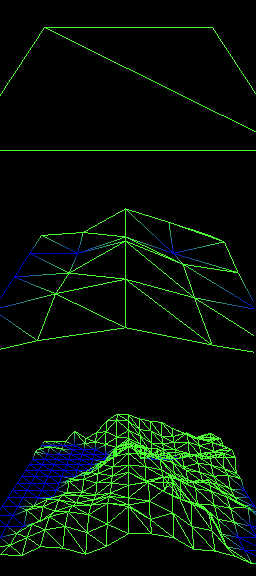Fractal terrain that is a full 2D surface (rather than 1D line) is created by making a grid of points, and applying the midpoint subdivision approach.

Sample code: mountain2d.py

# Fractal terrain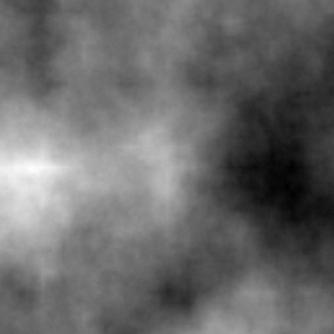A fractal heightfield can also be used for other purposes.

e.g.: clouds, noise textures

Sample code: heightfield.py

# Fractal terrain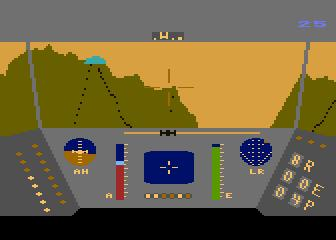Rescue on Fractalus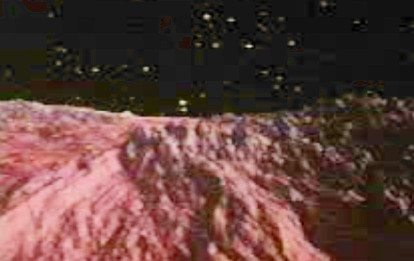Genesis EffectThis document is by Dave Pape, and is released under a Creative Commons License.